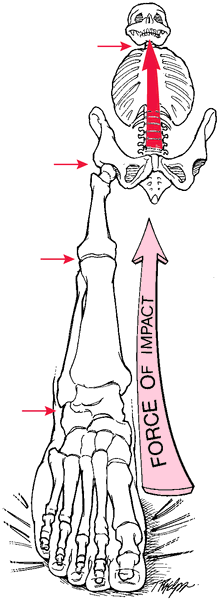electromotive force

(redirected from Induced emf)
Also found in: Dictionary, Thesaurus, Encyclopedia.

force

[fors]
energy or power; that which originates or arrests motion or other activity.Forces resulting from a fall are transmitted up to the spine through the long leg bones and pelvis. From McQuillan et al., 2002.
electromotive force the force that, by reason of differences in potential, causes a flow of electricity from one place to another, giving rise to an electric current.
reserve force energy above that required for normal functioning. In the heart it is the power that will take care of the additional circulatory burden imposed by bodily exertion.
shearing f's see shear.
van der Waals f's the relatively weak, short-range forces of attraction existing between atoms and molecules, which results in the attraction of nonpolar organic compounds to each other (hydrophobic bonding).

e·lec·tro·mo·tive force (EMF),

the force (measured in volts) that causes the flow of electricity from one point to another.

e·lec·tro·mo·tive force

(EMF) (ĕ-lek'trō-mō'tiv fōrs)
The force (measured in volts) that causes the flow of electricity from one point to another.

e·lec·tro·mo·tive force

(EMF) (ĕ-lek'trō-mō'tiv fōrs)
The force (measured in volts) that causes electricity to flow.
References in periodicals archive ?
Summed up, the iron-loss resistance depends on the rotor position, the quasi-rms value of the phase current and the induced EMF. So, in order to accurately determine the corresponding characteristics, the measurements should be taken for different values of all three variables within the respective ranges of interest.
Measurement of the induced EMF e by means of a search coil is impractical because it requires opening of the machine and mounting a search coil (an additional sensor), as described in Section III A.
In the shifting of slot openings method, the cogging torque was suitably reduced and the induced emf is better than other methods as seen in Table 3.
The experimental measurement of induced emf and power and cogging torque of DRFPMG prototype was carried out using a laboratory set-up shown in Figure 14.
Obtained induced EMF based on Faraday's law expressed by Equation (14) and flux linkage for different rotor angles and two different field currents 1 A and 5 A are presented in Table 1.
At first, an analytical method capable of calculating, with acceptable accuracy, flux distribution and terminal induced EMF characteristics, is applied to obtain the initial machine dimension, and the desired parameters before FEA is applied to complex magnetic axial flux path.
Section 2 introduces the general induced EMF derivation and verification.

Site: Follow: Share:
Open / Close